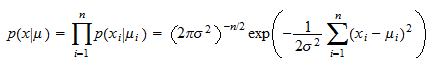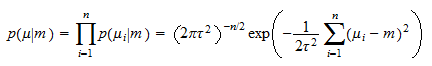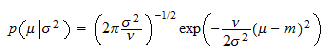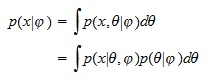StatLect

# Hierarchical Bayesian models

A hierarchical Bayesian model is a model in which the prior distribution of some of the model parameters depends on other parameters, which are also assigned a prior.## Definition

Given the observed data, in a hierarchical Bayesian model, the likelihood depends on two parameter vectorsandand the prioris specified by separately specifying the conditional distributionand the distribution.

In the literature it is often required that the likelihood does not depend on, that is,In this special case, the parameteris called hyper-parameter and the prioris called hyper-prior.

We use a broader definition of hierarchical model, that does not necessarily include assumption (1), because it allows for a unified treatment of several interesting models.

## Examples

The following examples illustrate two popular models that fall within our definition.

### Example 1 - Random means

Suppose the sampleis a vector of drawsfromnormal distributions having different unknown meansand a known common variance:Denote bythe vector of means:Conditional on, the observations are assumed to be independent. As a consequence, the likelihood of the whole sample, conditional on, can be written asNow, assume the meansare a sample of IID draws from a normal distribution with unknown meanand known variance, so thatFinally, we assign a normal prior (with known meanand variance) to the hyper-parameter:The model just described is a hierarchical model. With the notation used in the definition, we have,and the added assumption that### Example 2 - Normal mean and Gamma precision

Suppose that the sampleis a vector of IID drawsfrom a normal distribution having unknown meanand unknown variance.

The likelihood of the whole sample, conditional onand, isNow, assume that the meanis itself normal with known meanand variance, whereis a known parameter:Finally, we assign an inverse-Gamma prior to the parameter(i.e., a Gamma distribution to the precision):whereandare the two parameters of the Gamma distribution.

This is a very popular model, known as normal - inverse Gamma model.

It fits the above definition of a hierarchical model with,.

## Computations

The computation of the posterior distribution is usually performed in steps: firstis taken as given, and a conditional distribution foris derived; then a posterior foris computed.

The steps are as follows.

1. Conditional on(i.e., by keeping it fixed), compute:

1. the prior predictive distribution of:2. the posterior distribution of:2. By usingfrom step 1, compute:

1. the prior predictive distribution of:2. the posterior marginal distribution of:3. Compute the posterior joint distribution ofand:4. Compute the posterior marginal distribution of:When we are not able to carry out the integrations required to derive the predictive distributions, or when we cannot compute posteriors with Bayes' rule, then we can use other computational methods (e.g., the factorization method illustrated in the lecture on Bayesian inference). In these cases, the steps of the above procedure remain valid: we first derive posterior and predictive distributions given, by using whatever method is available to us; then, we use the conditional distributions thus derived to compute the posterior of.

## More than two levels

In the definition above, there were only two levels: a parameterand a hyper-parameter.

The definition can be generalized to more than two levels. For example, we could have a third parameter, the likelihoodand the priorwhich is specified by separately specifying the conditional distributions,and the distribution.

With more than two levels, the computation strategy is similar to that illustrated in the previous section. First, we take all parameters but one as given, and we derive the prior predictive distribution of, conditional on the parameters that have been kept fixed. Then, we use the predictive distribution thus obtained as likelihood, and we use it to obtain another prior predictive distribution for, conditional on a smaller number of parameters than in the previous step. And so on.